# Reducing Boolean Satisfiability to The Witness

## Spoiler Alert

This article contains major spoilers for The Witness. It goes into moderate detail about puzzle pieces.

## Background

Recently, I've been looking at Complexity Theory, which is a field in theoretical Computer Science. It concerns itself with how long algorithms take to run and how much space they use. It tries to classify problems as 'easy' or 'difficult' depending on the algorithms we have for solving them. In fact, it's far more textured than that. Problems form a hierarchy that relates the difficulties of seemingly disparate problems with one another.

You may have heard of the Travelling Salesperson Problem. That's classified as 'NP-hard'. How about checking if a number is prime? That's 'Polynomial'. What do these mean? Well, I'm not going to go into it here, but the point is that we can say with utmost confidence that solving the Travelling Salesperson Problem is inherently, a much more difficult problem than testing primality. We've connected seemingly unrelated things by weaving a fabric of complexity analysis between them. I find that fascinating.

## The Witness

So what's this article about? I'd like to try to weave that fabric a little further and take a look at Jonathan Blow's puzzles from The Witness. We'll go through something called a 'reduction', which is a method for transforming one problem into another. You can think of this as an algorithm that takes, as input, some representation of one problem and produces, as output, some other kind of problem.

Reductions are the threads that form this fabric. If we can find a reduction from problem A to B we can make claims about their relative difficulty. We can say that A cannot possibly be harder than B, because we can always just transform A into B and solve that instead. This does assume our reduction isn't too difficult to compute, otherwise we'd have to factor that in, too.

To be more specific, I'm going to demonstrate a reduction from a problem called 'Boolean Satisfiability', to puzzles from The Witness. This will give us an opportunity to see how reductions work in the context of something fun. When I first encountered reductions, I felt confused and didn't understand how people came up with them. This article is the walk-through I wish I'd had to build that intuition.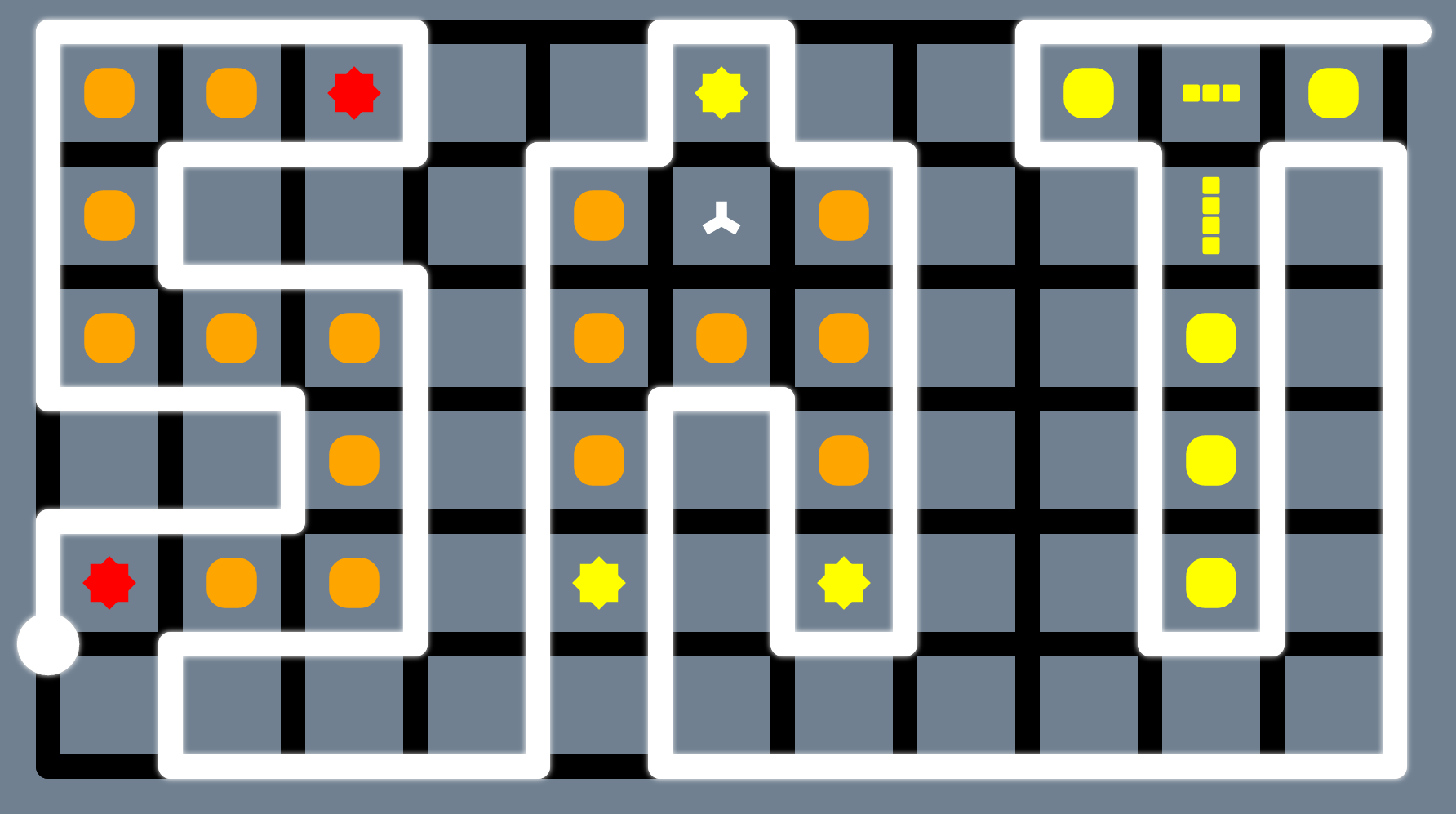Boolean Satisfiability is often abbreviated to 'SAT'

At this point, I should explain that if you are well-versed in Complexity Theory, this reduction would be a somewhat frivolous endeavour. The SAT problem (as it is often abbreviated) is a 'hard' problem and so by reducing SAT to The Witness, we're showing that puzzles from The Witness are at least as difficult as an already difficult problem. Not an earth-shattering result. The real charm would be to go in the other direction, but we're not going to do that today. Instead, we'll embrace the futility and frivolity.

## Getting Started

We should start by refreshing our memory on SAT.

SAT is the problem of finding a satisfying assignment for a boolean equation. In short, we need to come up with a true or false value for each variable in the equation to make the whole thing true. Here's an example problem:

(A v !B) ^ (!B v !C)

This can be read as '(A or not B) and (not B or not C)'. Let's try to solve this problem. What if we assigned A=true, B=true, C=true ? Well, that satisfies the first clause because (true or false) is true. How about the second? That's (false or false) which evaluates to false. Our attempt has failed.

Ok, so how do we fix it? How about A=true, B=true, C=false ? That works because each and every clause now evaluates to true and the equation has been satisfied. That wasn't so hard! Just a few moments ago I said this was a 'hard' problem. Well, this one was easy, but in general that's not the case. As we add more variables, the difficulty scales exponentially.

You may be wondering why we care about this problem in the first place. Why did we pick this? Well, clever people have already figured out how to reduce a plethora of other problems to SAT. Really important ones, too, like route planning and laying out circuit boards efficiently. In fact, SAT is depended on so heavily, there's an annual competition to see who can write the best solver. I expect to see your entry next year!

Ok, that's SAT out of the way. What about The Witness? Well, I'm not going to explain what all of the symbols mean here. If you're hazy on the details you can click on these to refresh your memory:SunElimination MarkTetris Block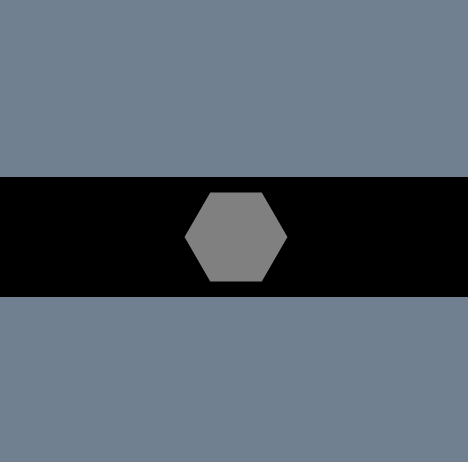Hexagon Dot

There are more puzzle pieces in the game, but this is just enough to do our reduction, so let's get on with that.

## The Reduction

So how do we do this? We need to come up with a method for transforming any SAT problem into a Witness puzzle. There should be a solution to the puzzle only if the SAT problem can be satisfied and there should be no solutions when it cannot. We somehow need to encode the structure of a generic boolean equation inside a Witness puzzle. So let's think about how we might do that.

The first thing to note is that each clause in a SAT problem is connected with an AND. Every one of these must evaluate to true for it to be a correct solution. This is vaguely reminiscent of the Sun symbols in The Witness. Each Sun must be paired up with another Sun of the same colour for there to be a solution. This is a bit tenuous, but maybe we can do something with this similarity.

Ok, what else do we know?

The way to solve a SAT problem is by choosing between true and false for each of the variables in the equation. There's a notion of 'choice'. What does it mean to 'choose' something in a Witness puzzle? What's the corresponding action for choosing? Well, the only influence a puzzler has over a Witness puzzle is to choose their path from start to finish. Do I go North, East, South or West at this junction?

So perhaps we could model variables as forks in the road that the puzzler must choose between. Taking the left fork could correspond to A=true and the right fork could be A=false. Now let's try to put this together with our Suns idea. Let's invent a red Sun to represent the first clause. In our SAT problem, if assigning A=true satisfies the clause, we should design our puzzle so that the Sun is paired up. If they choose to go right, the clause has not been satisfied so this should be an incorrect solution to the puzzle: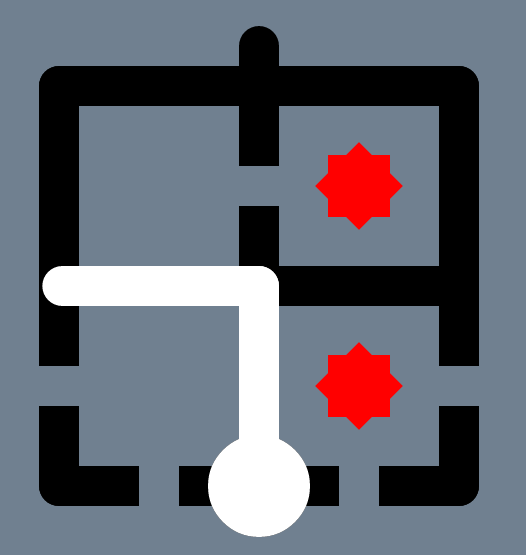Left Fork: A=true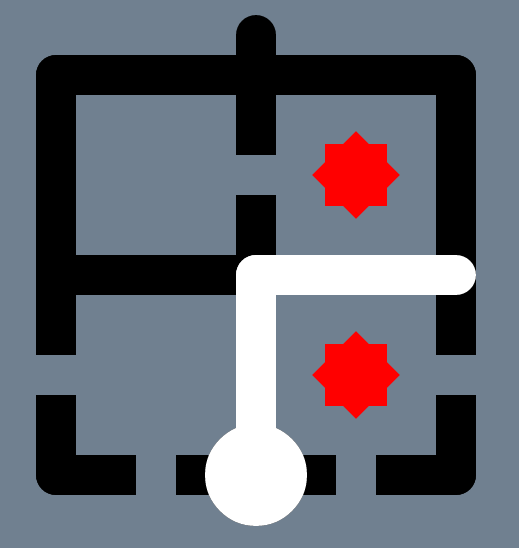Right Fork: A=false

So that's the basic idea. To recap, we're going take our SAT problem (whatever that may be) and turn it into a Witness puzzle. We're going to add a Sun for each clause and force the puzzler to make a choice as to whether to take a left fork or a right fork corresponding to a true or false assignment for each of the variables. We'll construct our puzzle based on which variables appear in which clauses and whether they are negated or not.

## Starting Small

Let's start by trying to create a puzzle for (A v B). This seems like the smallest possible step on the way to formulating a general method. We still have a single clause but we've added a variable. If we follow the rule of inventing a Sun for each clause in our equation, our puzzle should still only have a single colour Sun. What's different is the number of variables within that clause. There is now more than one way to satisfy this equation.

This means we need to build a puzzle that can be solved if either A=true or B=true. How do we do this? If we follow our rule that each variable is a fork in the road, we immediately hit an abundance of problems. Firstly, where do we put those forks? Do we stack forks on top of each other? Something like this: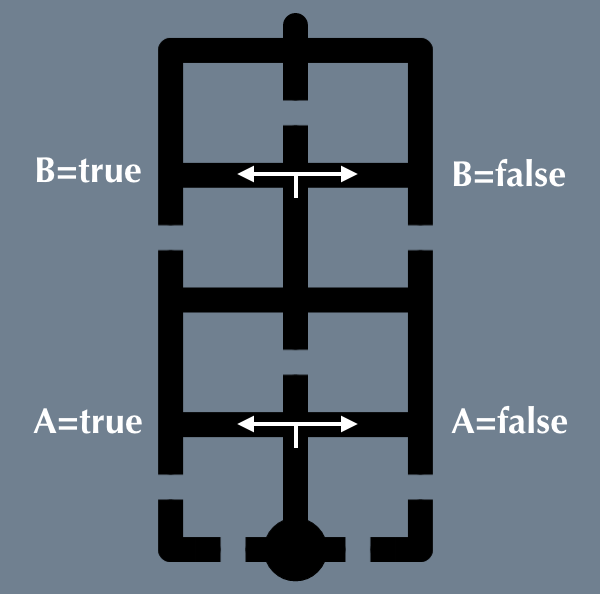Stacking forks on top of each other

If we go with that, how do we arrange our Suns? Let's make an arbitrary decision and place the Sun for the clause in the bottom-right corner. We then need to pair up this Sun if either A=true or B=true. This clearly isn't going to work. If we choose A=false, we've isolated our Sun so no matter how we choose B, we can't solve the puzzle. What's more, we're going to end up with three Suns in the puzzle and won't be able to pair them.

So how do we proceed? Let's think about our SAT problem again. We know that (A v B) is satisfied if either A or B is true. This is an 'at least one' relationship, i.e. at least one of the variables must evaluate to true for the clause to be true. Suns work a bit differently. Suns must be paired with 'exactly one' other Sun. Three or four just won't do! So if we can change these semantics from 'exactly one' to 'at least one' we may have a chance.

## 'at least one'

Let's restate our goal: We need to find a way to construct a Witness puzzle so that a Sun representing the clause can be paired with another Sun. We need to do so in a way that allows for any number of Suns of the same colour to appear in the puzzle. Blimey!

Perhaps Elimination Marks can help with this. Let's construct a puzzle with a Sun representing the clause placed in the bottom-right position. We'll then place 'at least one' other Sun in the puzzle and think about how we can use Elimination Marks to eliminate the excess Suns. The following puzzle isn't quite right, but it demonstrates what we're trying to do: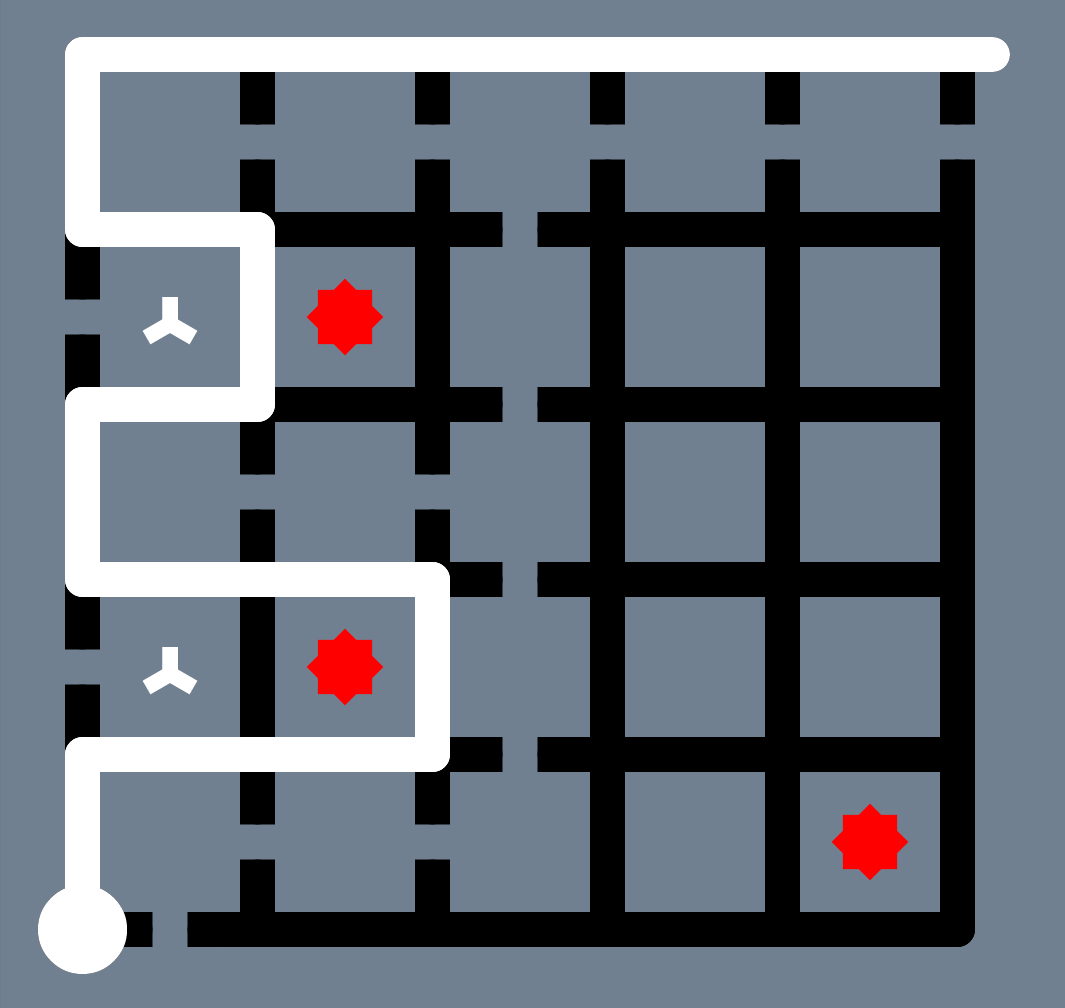Trying to pair up the bottom-right Sun

What's wrong with this approach? Well, we've successfully paired up the bottom-right Sun with one other Sun, but we've left an Elimination Mark dangling. There are no 'mistakes' in that 1x1 region for the Elimination Mark to mop up. Because of this, the path drawn above is not a correct solution.

What can we learn from this? Firstly, we should note that we can stack as many Suns on the left as we'd like, which means we're on the right track to meet our 'at least one' requirement. Secondly, we can choose which of the Suns to pair up depending on how we draw the path. Finally, we can force where the puzzler must go by simply removing sections of the path for them to travel.

I'd call that progress! Ok, so we haven't solved our problem yet, but we've learned a few things.

It seems that our next logical step is to see if we can find a way to get rid of those dangling Elimination Marks. Perhaps we can intentionally introduce mistakes to be eliminated. We'll have to be careful about how we do this as they should only really be mistakes under certain conditions. We're going to have to think this through.

## Intentional Mistakes

Before we dive into this, let's do a quick recap on how we got here:

We're trying to find a reduction from SAT to The Witness. We think we can use Suns to represent clauses which are satisfied by pairing each up with another Sun of the same colour. In SAT, clauses are satisfied if any of their variables evaluate to true. We need to mimic these semantics. We almost managed it with Elimination Marks, but ended up leaving a surplus in the puzzle. Now we're exploring whether we can fix this by introducing intentional mistakes for the Elimination Marks to eliminate. Are you still with me?

Ok, let's get to business.

We need to find a way for there to be a mistake in the same region as the Elimination Mark, but only if the Sun is paired. If it is not paired, the Elimination Mark should mop up the unpaired Sun, instead. Being an unforgiving kind of game, there are actually quite a few ways to introduce these kinds of mistakes. Here's one: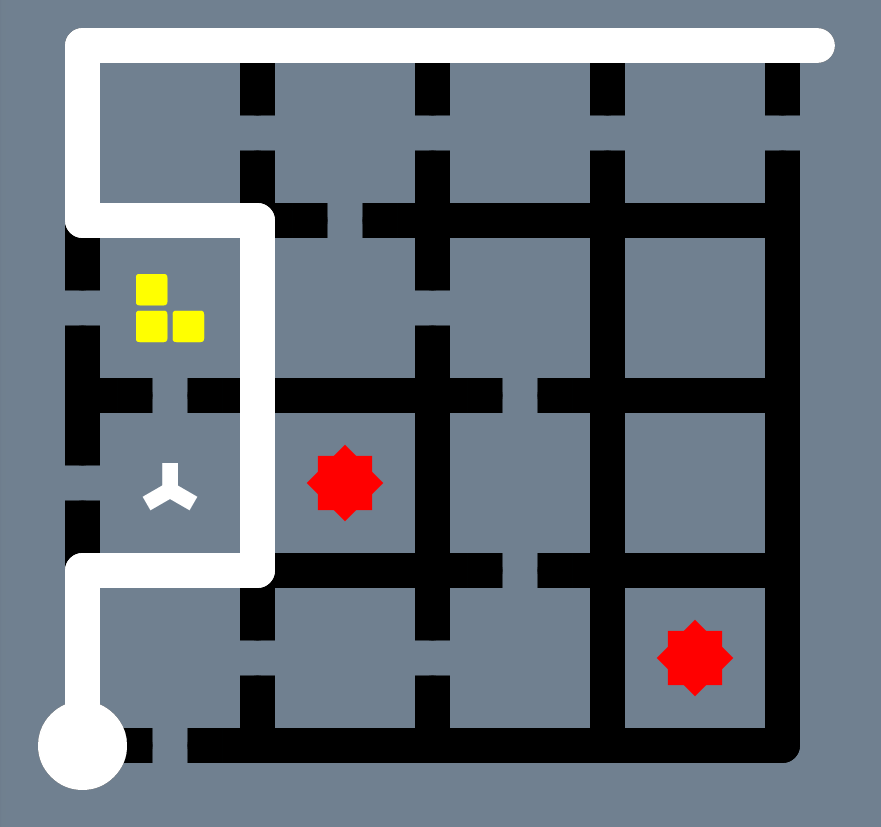Suns paired: Tetris Block is a mistake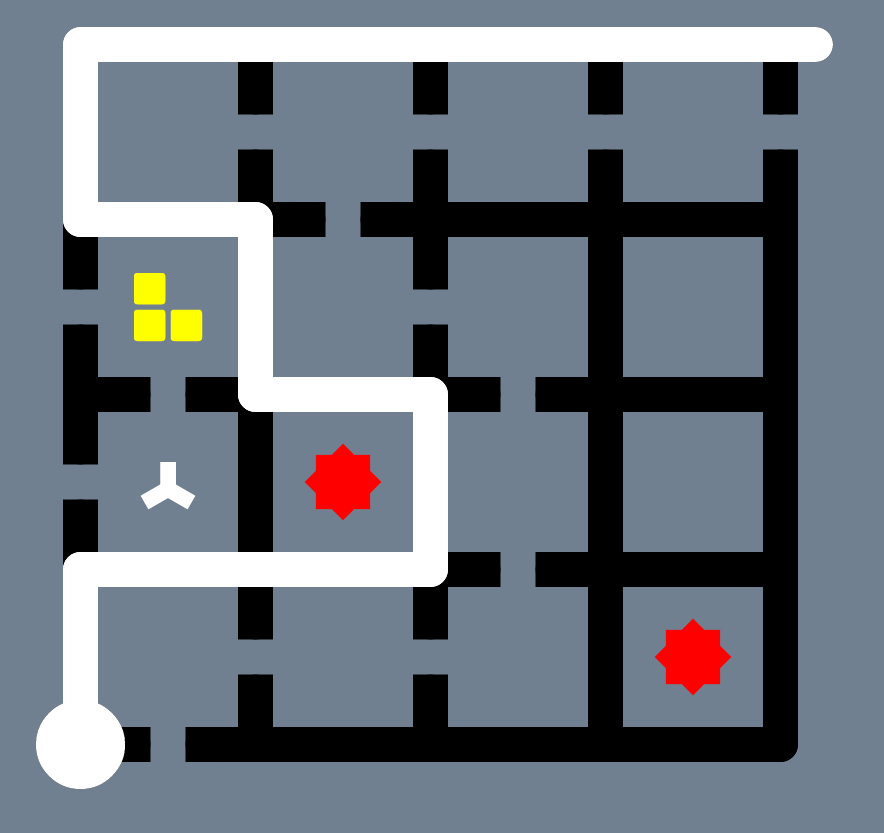Suns not paired: Sun is a mistake

In this case, only the puzzle on the left is correct, but the puzzler has the freedom to choose whether to pair the Suns or not. Tetris Blocks seem to lend themselves well to this. We can tailor their shape to match the region containing the unpaired Sun. This has the effect of toggling which thing is eliminated depending on which path is taken. Here are some alternatives that would work equally well: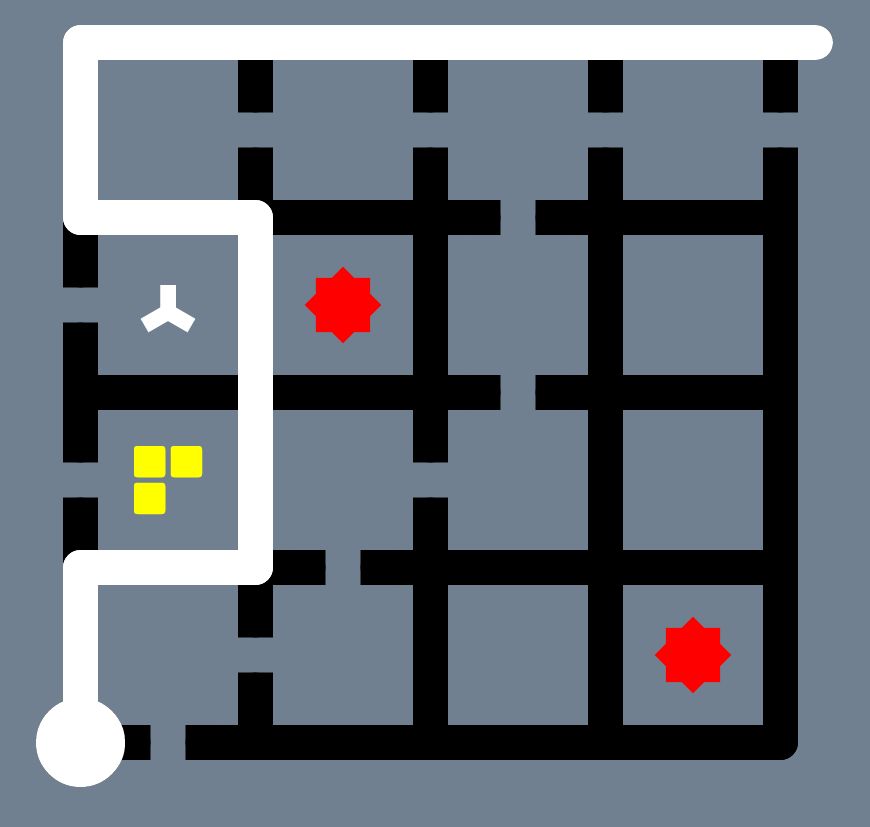Alternative 1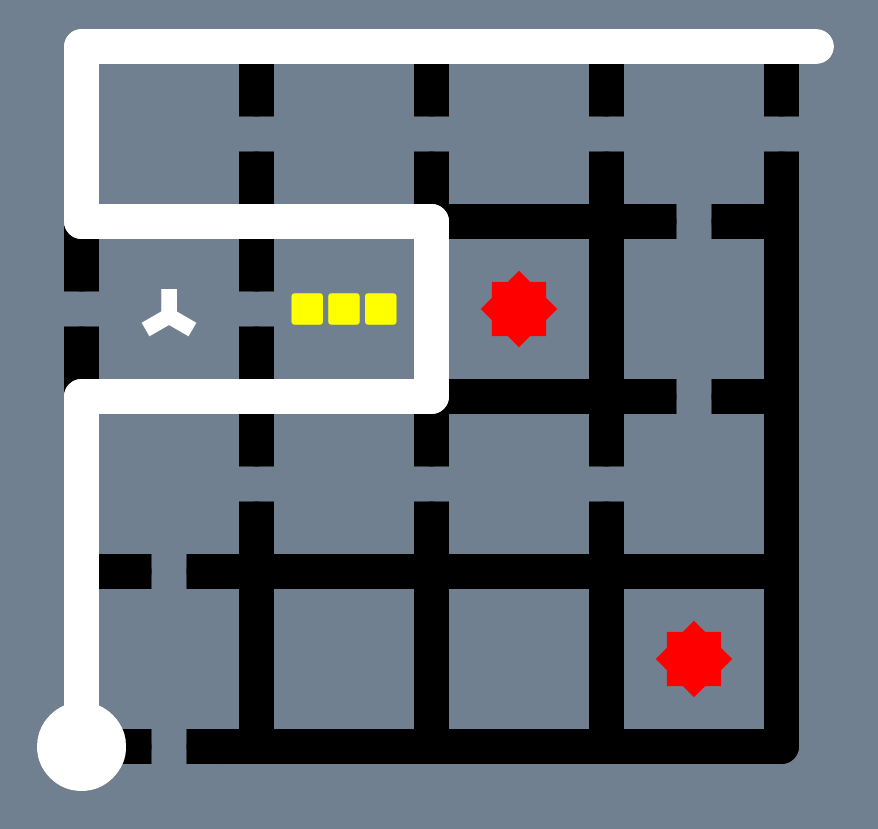Alternative 2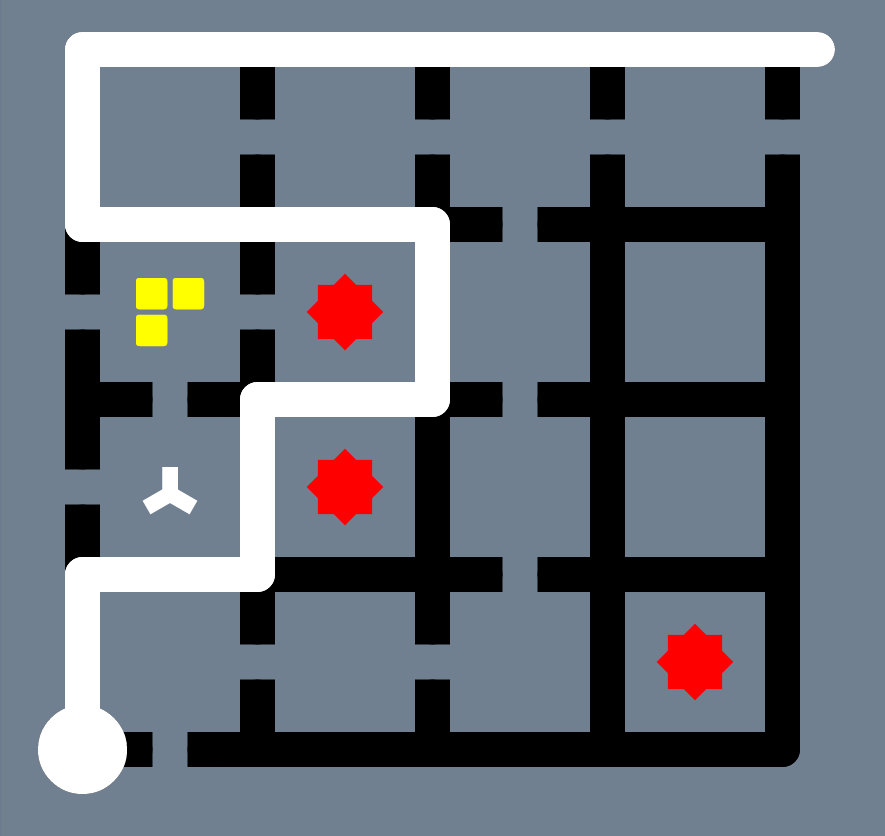Alternative 3

That last one's a bit different, but ponder it for a moment and you'll see that it works. In fact, we could have used some of the other puzzle pieces, too. So which of these should we choose? Let's defer that decision for later. Having a few options might help us if we get stuck.

## Freedom to Choose

We can now construct puzzles that contain as many Suns as we'd like and the puzzler has the freedom to choose which of those Suns are paired. Great! But how does this relate back to our original problem? We were trying to build a puzzle for (A v B). Are we there yet? Well, we haven't defined what it means to take one path or another. Does the following path mean A=false, B=true or is it just meaningless gibberish?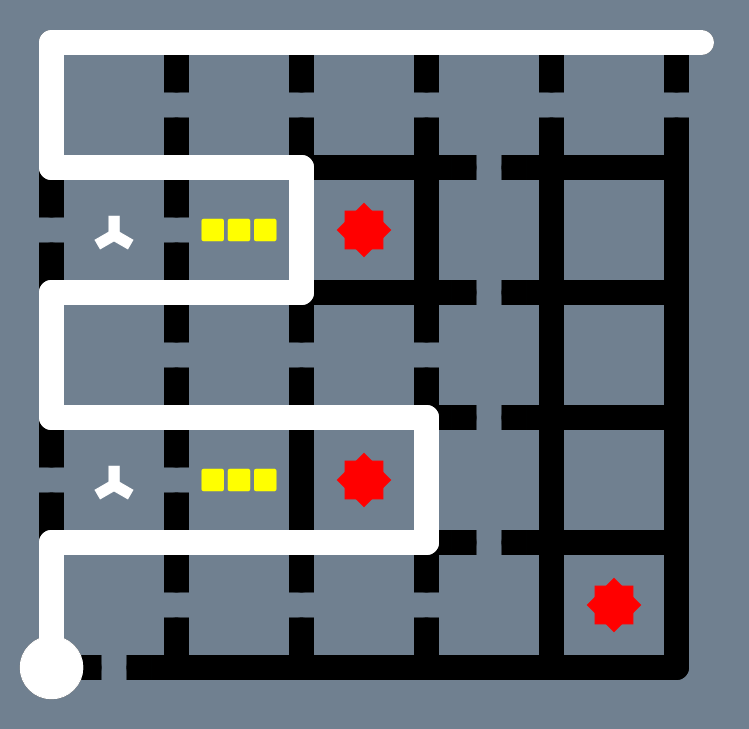Does this path mean anything?

But hold on a minute – we haven't really defined how a puzzle should be constructed for a given SAT problem. We assumed something like the above corresponds to (A v B), but is that really true? We began looking at this from the perspective of 'we have too many Suns, how do we cope with that?', but we're yet to tie this back to the original problem. What we really need are a few rules for how to build these puzzles, but so far we've only considered a single example. To proceed, we ought to consider a few more.

So let's cast our net a little further and consider (A) ^ (!A). I've left the brackets in so we can clearly see that this is made up of two clauses. Note that this problem is unsatisfiable. Every clause must be true and one of the two will be false depending on how we assign A. If you remember back, we kind of have a rule that each clause in our equation corresponds to a different colour Sun, but how do we deal with negations?

Well, we said that 'choice' corresponds to which path is taken through the puzzle. If the puzzler chooses a path that represents A=true, they should no longer be able to pair up Suns in cases where A is negated. Here is a simplified example that uses a Hexagon Dot to force the puzzler to make a choice.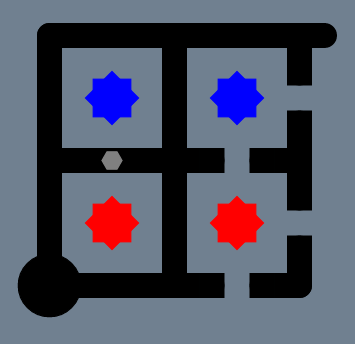Forcing a decision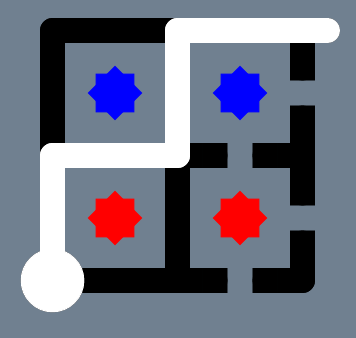A=true, red Suns paired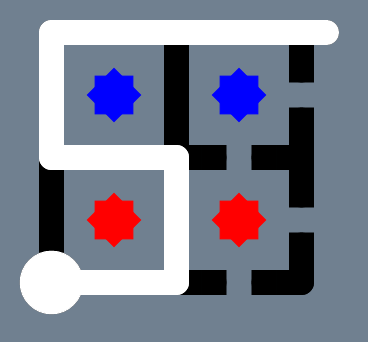A=false, blue Suns paired

Earlier on, we talked about using 'forks in the road' to force the puzzler to choose how to assign true or false to each variable. That's exactly what we're doing here, although it's not as obvious. The fork is a decision between North and East from the start position. The Hexagon Dot insists that one or the other is chosen, otherwise they could go straight up the left-side, which would correspond to assigning both true and false to A.

## Putting it Together

So now we're ready to start putting things together. Let's try to do this gradually and construct a puzzle that represents (A v !A). This is a single-clause, single-variable problem, but it contains a negation. We know that a single clause means we put a Sun in the bottom-right corner. We also know that we should add our special combination on the left for each occurrence of a variable, so let's do that: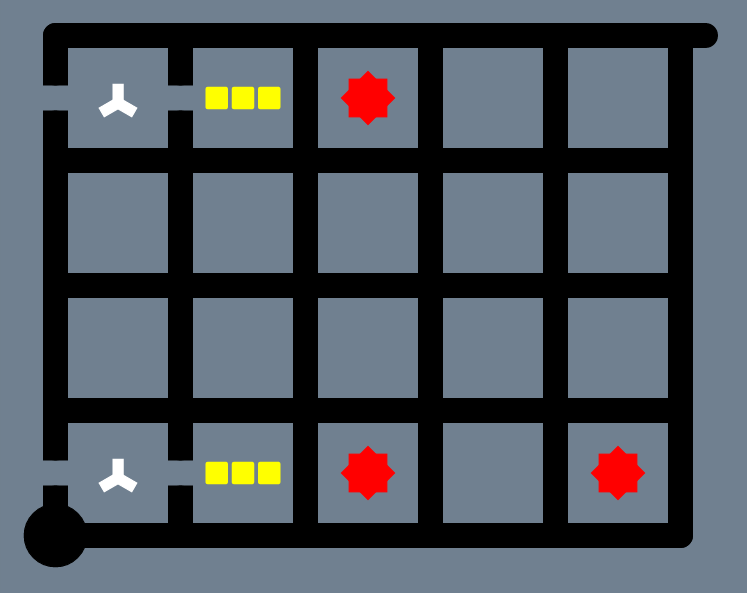Putting it together: Part 1

We then need to force the puzzler to choose whether to assign A=true or A=false. We can do this by adding a Hexagon Dot along a path that runs between the symbols and an alternate path along the right-hand side. At this point, we also need to remove other sections of path: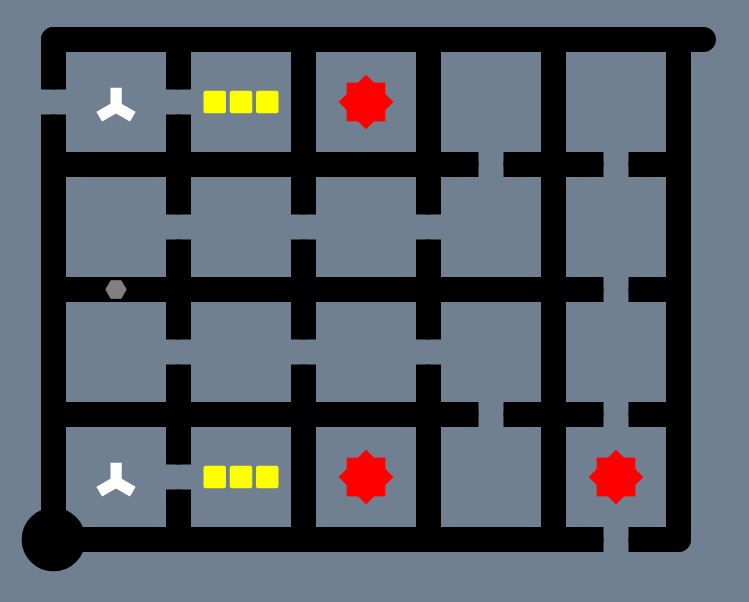Putting it together: Part 2

And that's it... or is it? The eagle-eyed amongst you may have noticed a problem with this puzzle. We'll get to that in a moment. Before then, let's see what paths corresponding to A=true and A=false look like: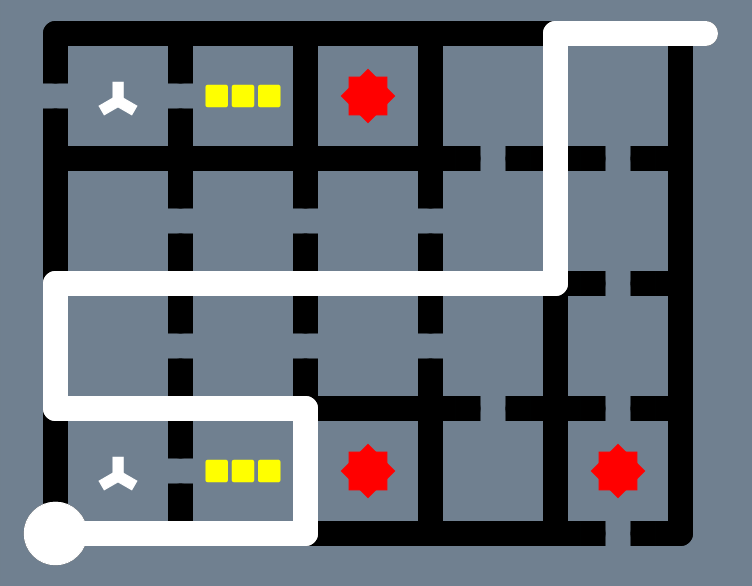Path for A=true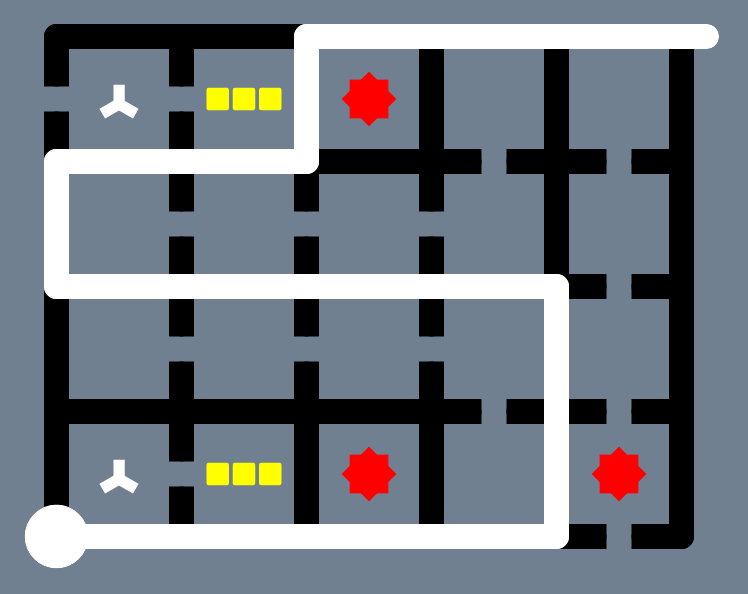Path for A=false

You can see that the Sun that's paired is different in each case. We've arbitrarily defined the bottom Sun to mean A=true and the top to mean A=false, but we could flip this if we wanted. The Hexagon Dot we placed down the middle forces the puzzler to choose between one or the other.

Ok, so what's this problem I mentioned? Well, when we figured out our combination of symbols, we hadn't accounted for the case where the entire region is skipped. We'd only anticipated a single 1x1 region. There are now two mistakes in that region: the Tetris Block and the Sun. This is one too many for our Elimination Mark to mop up. We've found a bug!

## Fixing the Bug

Fear not! When we introduced intentional mistakes to the puzzle, we had a few alternatives. If we can find one that's valid when contained within the skipped region, we're golden. Let's remind ourselves of the other ways to introduce intentional mistakes. Which door should we choose?Door number 1Door number 2Door number 3

There are goats behind two of these doors. Can you figure out which has the sports car?

Ok, enough of that. The right answer is Door number 3. This is the only pattern where the Tetris Block is valid when the bottom-right Sun is paired up. When the region is skipped, the Tetris Block becomes invalid, but the Suns within that skipped region are paired, so there is only one mistake, rather than two. This is very confusing! Perhaps if we see how it works in different contexts, that will help: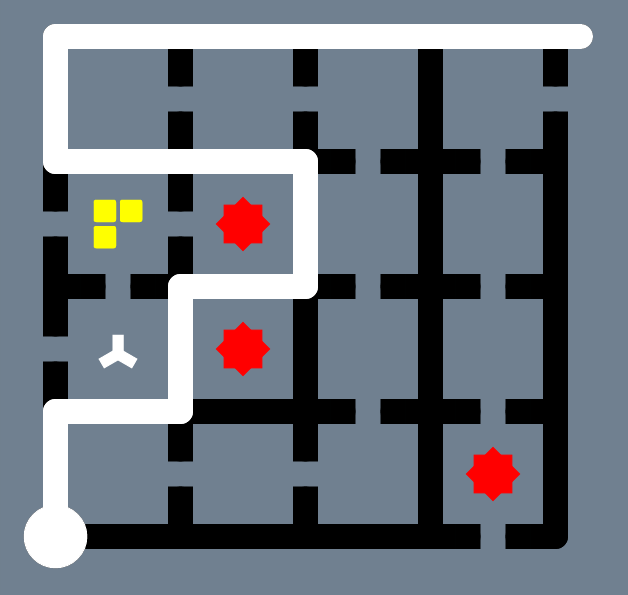Suns paired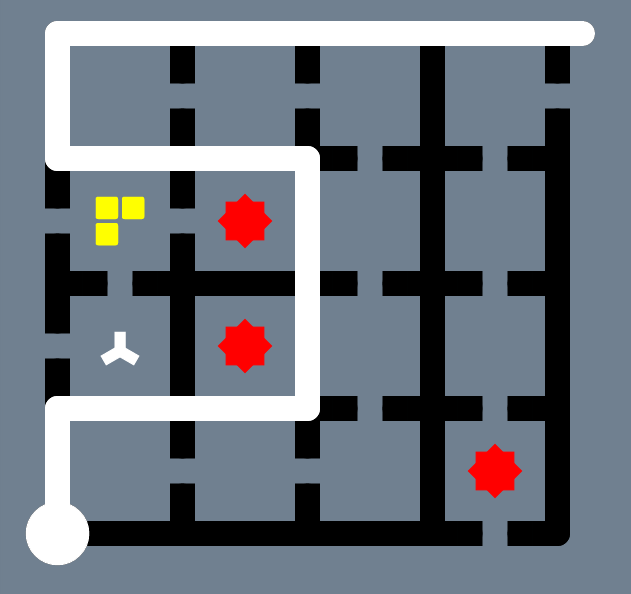Suns not paired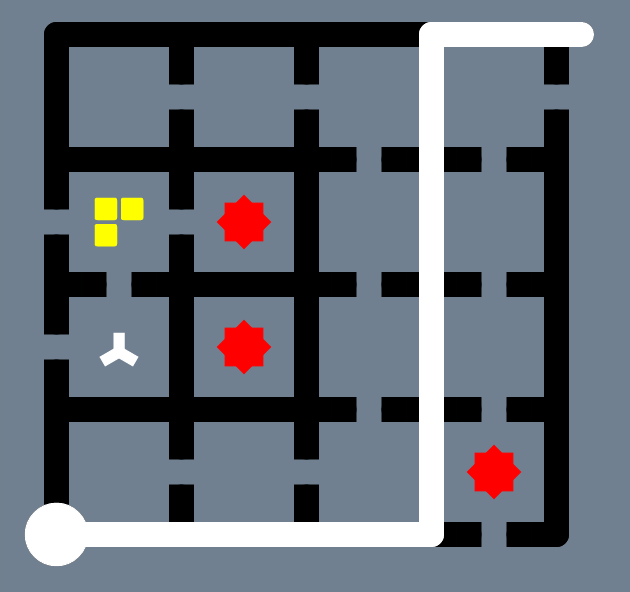Suns skipped

## Some Clarification

At this point you might be wondering what the difference is between 'Suns not paired' and 'Suns skipped'. Let's briefly consider the example (A v A) so that I can clarify: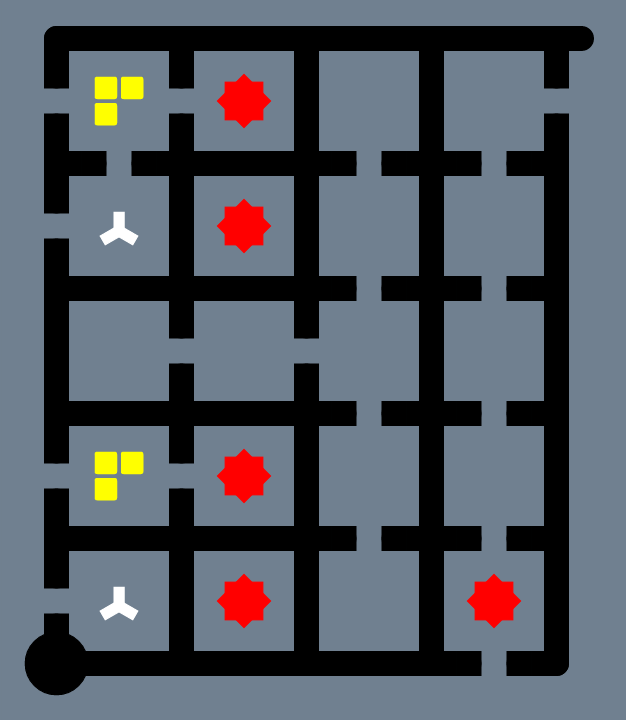The puzzle corresponding to (A v A)

The puzzle above is the same as the (A v !A) example we looked at earlier except we're now using the new pattern of symbols. We've also removed the Hexagon Dot running down the middle as we've dropped the negation. If we choose the path where A=true, that means we follow the left-hand side of the puzzle, up through the symbols. If we choose A=false, that leads alongside the symbols, skipping those regions.

If we consider the case where A=true, we actually have a choice in which Sun is paired. Do we pair one at the top or the bottom? Both are valid. This actually corresponds to our equation: (A v A). If we choose the bottom Sun, that's kind of like saying 'use the first A to make the clause true' and the top one relates to the second A. Let's see what these cases look like, as well as the case for when A=false: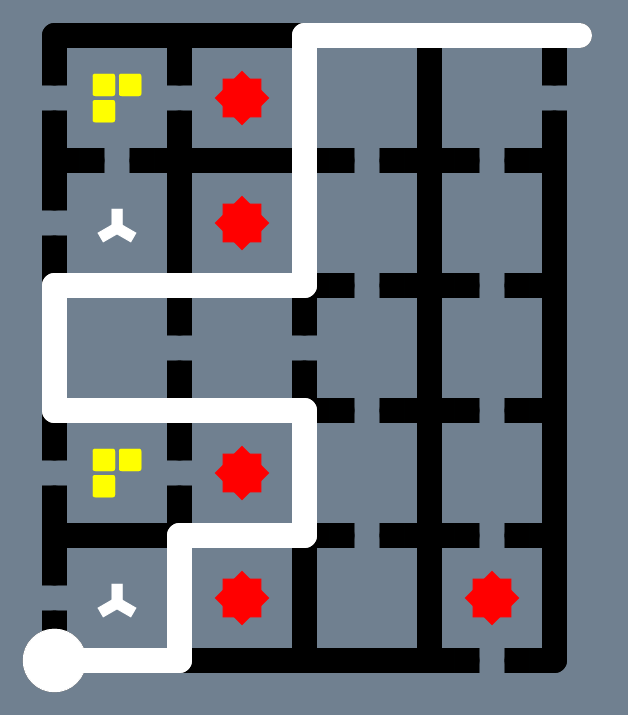A=true, bottom Sun paired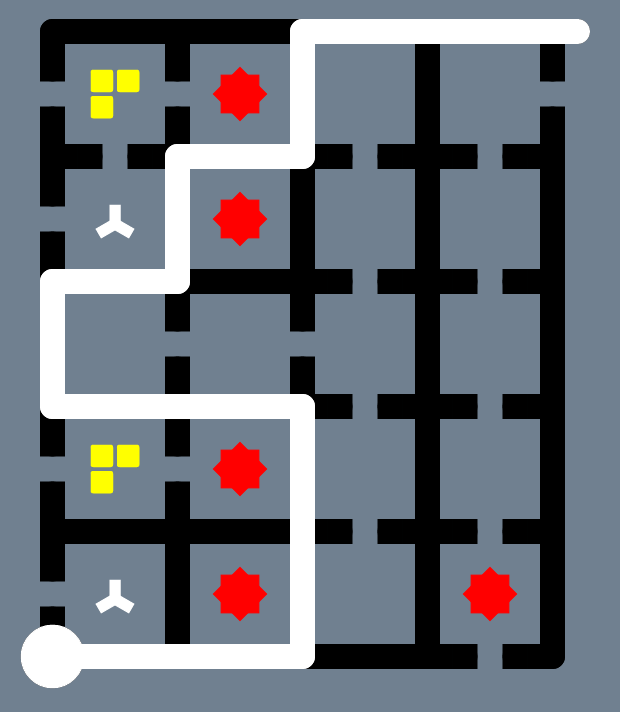A=true, top Sun paired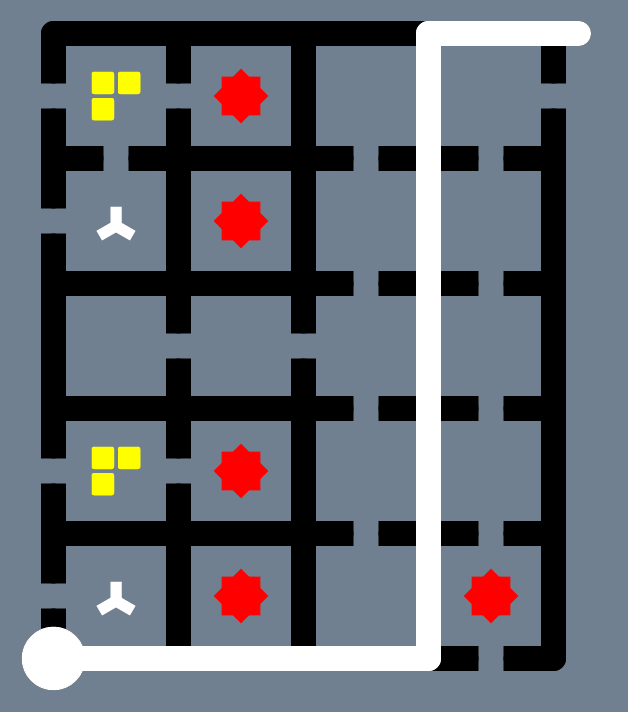A=false, neither paired

Notice that the two on the left are both genuine, correct solutions for the puzzle, whereas when A=false, the bottom-right Sun has not been paired up making it an incorrect solution.

## General Method

We're now reaching the end of our journey and are ready to formulate our general method. When we put everything together, we end up with a process that looks something like this:

• 1. Place a uniquely coloured Sun bottom-right for each clause
• 2. For each variable:
• a. For each clause containing the variable (as a positive):
• i. Add an Elimination Mark, Tetris Block and Sun to the left of the puzzle
• b. Add an alternative path that runs alongside this section
• c. Re-combine this path with the main path and add a Hexagonal Dot
• d. Repeat a, b for the case where the variable is negated
• 3. Make the rest of the puzzle unreachable by removing sections of path

If this sounds complicated, that's because it is! There's a lot going on there, so maybe a concrete example will help. Let's end where we started and build the puzzle corresponding to (A v !B) ^ (!B v !C). I have tried to annotate this puzzle as best I can, but it's still incredibly large and takes a long time to grok: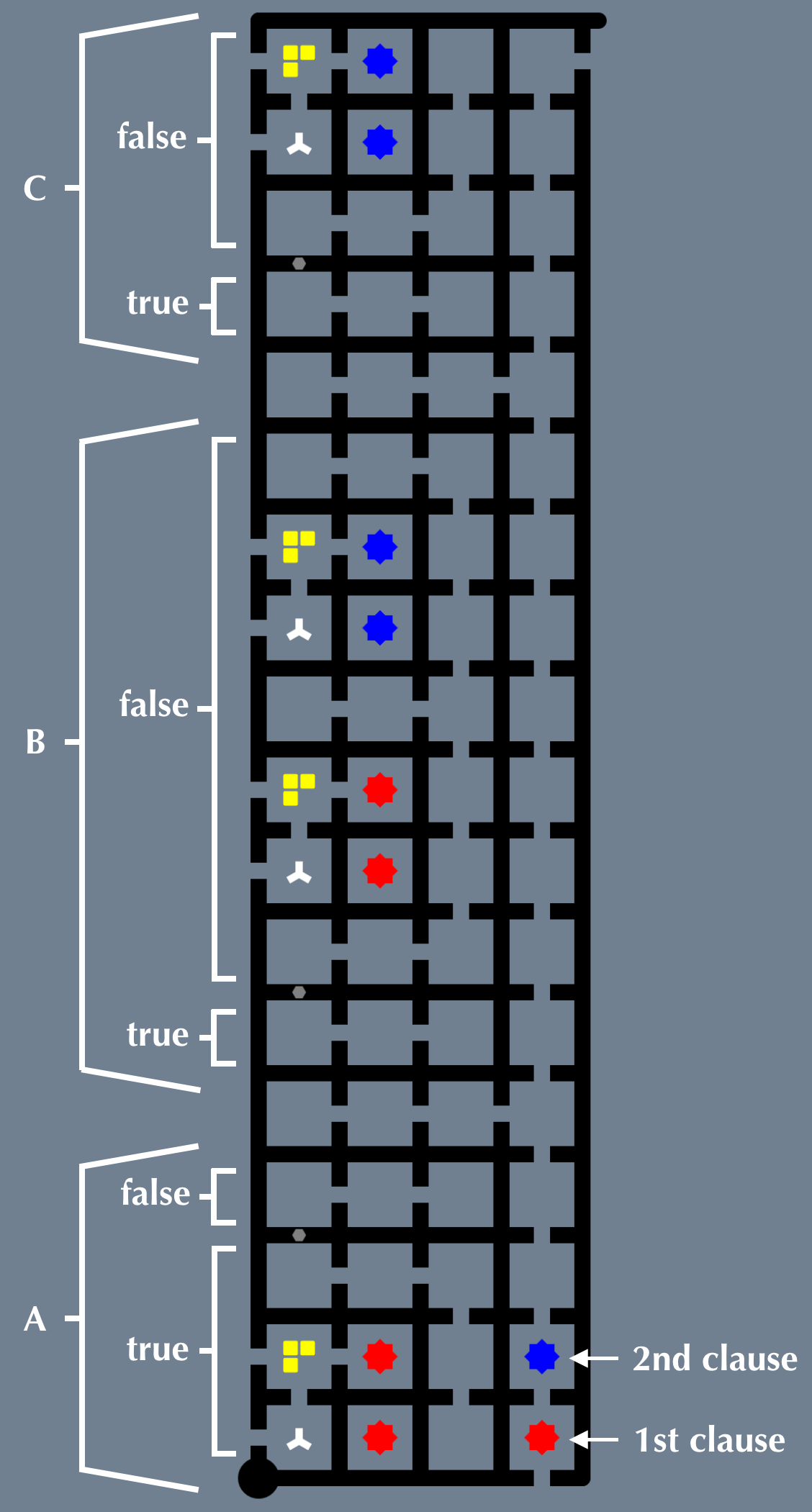The SAT problem (A v !B) ^ (!B v !C) represented as a puzzle from The Witness

The puzzle above is the result of reducing our SAT problem to The Witness. The method outlined above is the 'reduction' we've been seeking. It's quite amazing how we started with a few simple observations and by careful reasoning we've ended up with something incredibly complex. It's startling just how large the puzzle is for such a simple problem. That tends to be how reductions go.

Towards the start of this article, when talking about difficult, I said:

This does assume our reduction isn't too difficult to compute, otherwise we'd have to factor that in, too.

Let's consider this for a moment. We could write a program that performs this reduction. How 'expensive' would it be to compute? Well, although we end up with a huge problem, building the puzzle isn't all that difficult. Each occurrence of a variable adds roughly 18 cells to the grid and that doesn't change whether we have three variables or a million. That means we could build this puzzle in linear time by reading through the equation.

Finally, here are a couple of correct paths through the puzzle: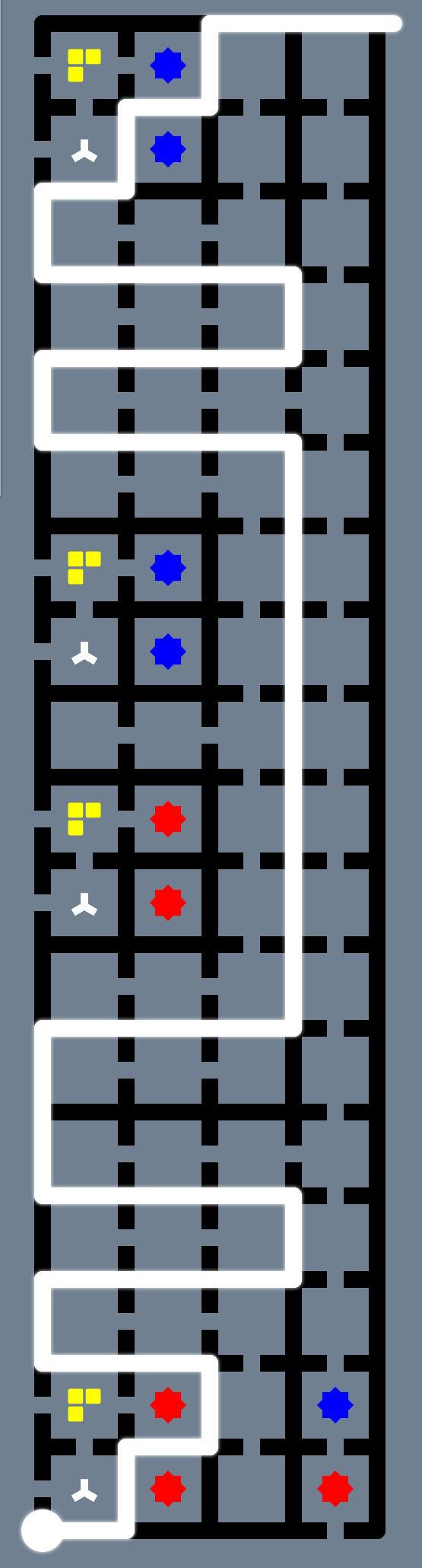Path for A=true, B=true, C=false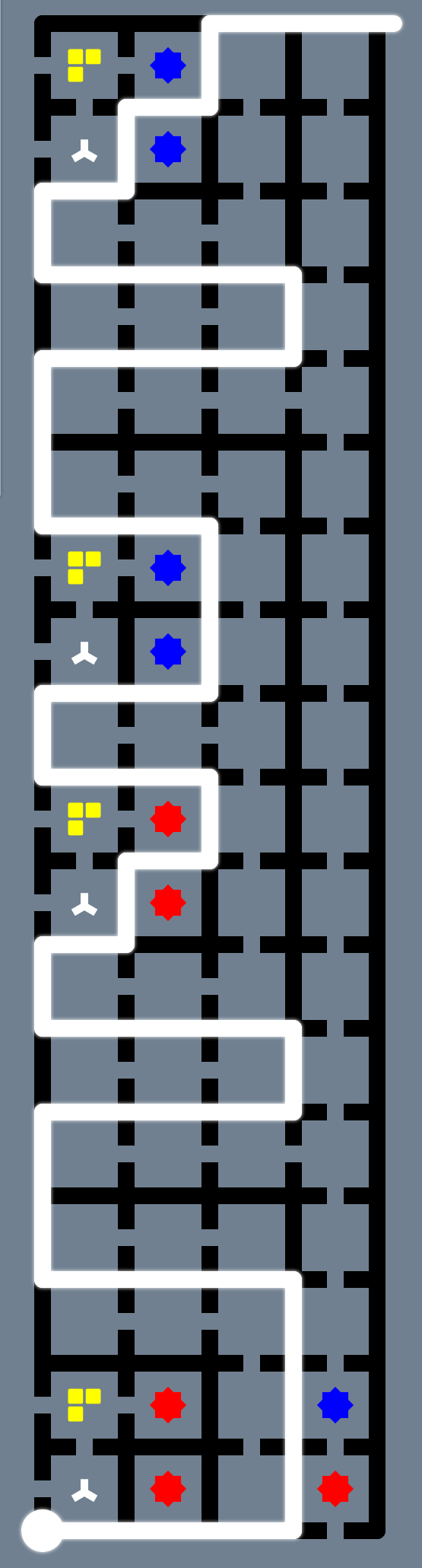Path for A=false, B=false, C=false

There's an interactive version of this puzzle here. If you decide to try this, see if you can figure out the corresponding assignments of A, B and C for your path.

## Closing Words

Hopefully this has given you some intuition for how reductions work. This is arguably a lot more complicated than most, but perhaps more interesting, too. One of the wonderful implications of all this is that you could solve all kinds of problems like route planning by reducing them to fiendishly difficult Witness puzzles. Those puzzles would probably be so large they'd reach the moon!

If this article has piqued your interest, you may wish to try writing a program to do this reduction. I'll happily add links to code or screenshots to this section if anyone is determined enough to try it. If the reduction in the other direction seems tempting, that's something I'd like to look at in coming months. I don't think it's possible for reasons I won't go into here.

You may be interested to hear that I'm writing a programming language called 'Sentient' that deals with these kinds of problems. It's still in alpha, but you can find the website here. Finally, I'd like to thank Tom Stuart for encouraging me to put pen to paper. I'd also like to thank Matthew Gruen for building The Windmill, which I have used extensively for taking screenshots.